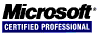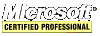# PHP Quick Tutorial

## Including Other Files

Include a header and footer page for a website using the `include` keyword. PHP exits PHP mode when a file is included using `include`.

``````<!-- header.html -->
<html>
<title>PHP INCLUDE EXAMPLE</title>
<body>
``````
``````<?php
// echo_output.php
echo \$output;
?>
``````
``````<!-- footer.html -->
</body>
</html>
``````
``````<?php
\$output = `ls -al`;
echo '<pre>';
include "echo_output.php";
echo '</pre>';
include "footer.html";
?>
``````

## Functions

PHP has a huge library of built-in functions. This tutorial does not cover everything.

### Variable Functions

Use the `isset` function to determine if a variale is set to a value.

``````<?php
\$string;
\$set = isset(\$string);
echo "\\$string is set? \$set";
?>
``````

Use the `unset` function to free the memory the variable is using.

``````<?php
\$string = 'how now brown cow';
unset(\$string);
?>
``````

Use the `var_dump` function to dump information out about a variable or array.

``````<?php
\$string = 'how now brown cow';
var_dump(\$string);
?>
``````

### String Functions

The `strlen` function returns the length of a string.

``````<?php
\$string = 'how now brown cow';
\$len = strlen(\$string);
echo \$len;
?>
``````

To remove the whitespace at the beginning and end of a string, use `trim`.

``````<?php
\$string = '	 how now brown cow	 ';
\$string = trim(\$string);
echo \$string;
?>
``````

To remove the whitespace at the beginning of a string, use `ltrim`.

``````<?php
\$string = '	 how now brown cow';
\$string = ltrim(\$string);
echo \$string;
?>
``````

To remove the whitespace at the end of a string, use `rtrim`.

``````<?php
\$string = 'how now brown cow 	 ';
\$string = rtrim(\$string);
echo \$string;
?>
``````

Use `str_replace` to replace text in a string. It takes three parameters: the text to search for, the text to replace it with, and the string to work with. It is case sensitive. The case insensitive version is `stri_replace`.

``````<?php
\$string = 'how now brown cow';
\$string = str_replace('cow', 'dog', \$string);
echo \$string;
?>
``````

To replace just a single character in the string use the curly braces `{}`.

``````<?php
\$string = 'how now brown cow';
\$string{0} = 'H';
echo \$string;
?>
``````

Extract part of a string using `substr`. The first parameter is a string, the second is the start position, and the third is the length which is optional.

``````<?php
\$string = 'how now brown cow';
echo substr(\$string, 4);
echo substr(\$string, 4, 3);
?>
``````

Use `strpos` to find the position of a substring inside a string. It returns `false` if the substring is not found.

``````<?php
\$string = 'how now brown cow';
\$i = strpos(\$string, 'cow');
if(\$i === false)
else
echo "cow at position \$i";
?>
``````

### Array Functions

PHP makes working with arrays a breeze with functions that can sort, filter, shuffle and intersect them.

To return the number of elements in the array, use the `count` function.

``````<?php
\$arr = array(1, 2, 3, 4, 5, 6);
\$c = count(\$arr);
echo \$c;
?>
``````

To strip all the duplicate values from an array, use the `array_unique` function.

``````<?php
\$arr = array(0, 0, 1, 2, 3, 3, 4, 5, 6, 6);
\$arr = array_unique(\$arr);
foreach(\$arr as \$i) {
echo \$i;
}
?>
``````

To randomize the order of the elements in an array, use `shuffle`. `shuffle` does not return a value.

``````<?php
\$arr = array(0, 1, 2, 3, 4, 5, 6);
shuffle(\$arr);
foreach(\$arr as \$i) {
echo \$i;
}
?>
``````

To determine if a value is in an array, use `in_array`.

``````<?php
\$arr = array(0, 1, 2, 3, 4, 5, 6);
\$found = in_array(3, \$arr);
if(\$found === true) {
echo '3 is in the array';
}
else {
echo '3 is not in the array';
}
?>
``````

To create a new array based on the keys of an existing array use `array_keys`.

``````<?php
\$arr = array('color1' => 'red', 'color2' => 'green', 'color3' => 'blue', 'color4' => 'orange', 'color5' => 'yellow', 'color6' => 'black', 'color7' => 'pink');
\$keys = array_keys(\$arr);
foreach(\$keys as \$key => \$value) {
echo "\$key = \$value\n";
}
?>
``````

To create a new array based on the values of an existing array use `array_values`.

``````<?php
\$arr = array('color1' => 'red', 'color2' => 'green', 'color3' => 'blue', 'color4' => 'orange', 'color5' => 'yellow', 'color6' => 'black', 'color7' => 'pink');
\$keys = array_values(\$arr);
foreach(\$keys as \$key => \$value) {
echo "\$key = \$value\n";
}
?>
``````

To sort an array use `asort` to sort by the array values and `ksort` to sort by the array keys. To reverse sort use `arsort` and `krsort`, respectively. These function do not return a value as they do their work in place.

``````<?php
\$arr = array('color3' => 'red', 'color2' => 'green', 'color1' => 'blue', 'color0' => 'orange', 'color5' => 'yellow', 'color6' => 'black', 'color4' => 'pink');
foreach(\$keys as \$key => \$value) {
echo "\$key = \$value\n";
}
asort(\$arr);
foreach(\$keys as \$key => \$value) {
echo "\$key = \$value\n";
}
ksort(\$arr);
foreach(\$keys as \$key => \$value) {
echo "\$key = \$value\n";
}
?>
``````

To turn array elements into variables, use the `extract` function. It takes three parameters: the array to extract, a constant telling how to prefix the variables, and the prefix to give the name of the variables. `extract` does not return a value. The second parameter must be one or more of the following.

• EXTR_OVERWRITE: if the variable exists already then overwrite it.
• EXTR_SKIP: if the variable already exists then skip it moving on to the next one.
• EXTR_PREFIX_SAME: if the variable already exists then use the prefix specified in the third parameter.
• EXTR_PREFIX_ALL: prefixes all variables with the prefix specified in the third parameter regardless of whether the variable exists already.
• EXTR_PREFIX_INVALID: uses a prefix only if the variable name start with a number.
• EXTR_IF_EXISTS: extracts only variables that already exist.
``````<?php
\$arr = array('color3' => 'red', 'color2' => 'green', 'color1' => 'blue', 'color0' => 'orange', 'color5' => 'yellow', 'color6' => 'black', 'color4' => 'pink');
extract(\$arr, EXTR_PREFIX_ALL, 'arr');
echo \$arr_color3;
echo \$arr_color2;
echo \$arr_color6;
?>
``````

### User Defined Functions

With functions, organized and reusable code can be created. They make code more readable and without them it is not possible to write easily maintainable code because there are identical blocks of code in multiple places.

Declare a function with the `function` keyword followed by the name of the function and any parameters are inside parentheses. Here is a function declared with a single parameter. Two functions cannot share the same name.

``````<?php
function square_it(\$number) {
return \$number * \$number;
}
\$value = 2;
\$squared = square_it(\$value);
print \$squared; // prints 4
?>
``````

Here is a function declaration with two parameters.

``````<?php
return \$num1 + \$num2;
}
\$val1 = 3;
\$val2 = 4;
?>
``````

Non-object values being passed into a function are passed by value not by reference. Use the & symbol to pass a variable by reference.

``````<?php